Phonon Echoes in a Glass at Low Temperatures

Sunil Sandhu March 24, 2007

(Submitted as coursework for AP272, Stanford University, Winter 2007.)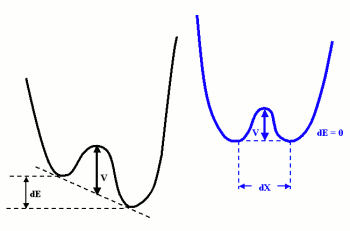Fig. 1: Schematic drawing of possible two-well potential configuration in glass with potential barrier (V) and generalized coordinate difference (dX). dE is a measure of energy asymmetry .

In various physical systems there has been experimental observation of the coherent effect known as echo. Examples are photon echoes from optical transitions in solids, magnetostatic echoes in yttrium iron garnet, cyclotron echoes in plasma, echoes from molecular transitions in gas, spin echoes, and phonon echoes in piezoelectric crystals and amorphous solids. Generally, all echo phenomena can be divided into two types of systems depending on the non-linear mechanism responsible for echo formation [1,2]. In an "anharmonic-oscillator" interaction system, the source of echo formation is the nonlinear coupling between different oscillator modes, or, alternatively, a single anharmonic mode of oscillation. On the other hand, in a "parametric field-mode" interaction system, an applied field couples to the previous excited modes of the system resulting in phase-reversal and echo formation. The latter system is the source of phonon echo formation in certain amorphous solids (e.g. glass). Phonon echoes generated in glass at low temperatures, besides manifesting the existence of intrinsic two-level configurational states within glass, provide a powerful probe of the dynamics (e.g. relaxation time, deformation potential) of these two-level systems at low temperature [3,4]. The first experimental study of phonon echoes in a structurally disordered solid, fused silica glass was by Brage Golding and John E. Graebner in 1976 .

Modelling Defects in Glass at Low Temperature

The large acoustic absorption in acoustic experiments involving glass at low temperature suggest a strong coupling between strain and some type of relaxation defect possessing a distribution of relaxation times much broader than that found for defects in crystalline solids. A phenomenological theory to explain this behavior introduced a broad distribution of two-level systems that are assumed to be an atom or a group of atoms residing in a double-well potential (Figure 1) . The random distribution of energy asymmetry, barrier height (V), and generalized-coordinate separation distance (dX) in the disordered system results in a continuous distribution for the tunnelling energy (E) between the two lower energy levels. At temperatures comparable to the barrier height (kT ∼> V), the atoms are thermally excited over the barrier. At low temperatures (kT << V), appreciable atomic displacement is impossible . However, the continuous distribution of E allows transitions from one well to another to take place by phonon assisted tunnelling where the phonon has a frequency corresponding to E . This tunneling through the potential barrier results in the mixing of the eigenstates of the two independent potential wells resulting in an energy splitting E.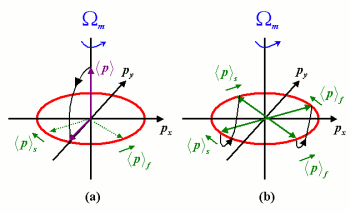Fig. 2: (a) Vector diagram showing the result in the rotating frame of applying a π / 2 pulse to the pseudopolarization vector

.

f and

s mimic the dephasing effect. (b) Vector diagram showing the result of application of a following π pulse to

. The dephasing effect is reversed resulting in the coherent formation of an echo.

This two-level model helps to explain several characteristics of glass at low temperatures. For example, the observed lowering of the the thermal conductivity of glass at low temperatures is due to resonant absorption of thermal phonons by the two-levels resulting in a lowering of the thermal phonon mean free path . Another characteristic is the observed saturation behavior of glass at low temperature which is consistent with the behavior of two-level systems where, in general, if enough energy is pumped into the system equalizing the thermal populations of the two levels, the system can extract little additional energy from the driving field. This saturation feature is observed in the phonon echo experiments below. One other important effect of low temperatures is that the phase memory time of these two-level systems becomes extremely long allowing for coherent effects like photon echoes and self-induced transparency to be observed [4,5]. In fact, the clearest indication of the increase in phase memory time is given by the observation of phonon echoes .

Theory of Phonon Echoes in Glass

In the two-level system model for glass, we can define a pseudopolarization vector (p) analogous to the magnetization vector of a system of spins  or the pseudo-electric dipole moment used in modeling coherent effects arising from optical transitions . The transverse components of the expectation value of the pseudopolarization vector are related to the components of stress which are in phase and out of phase with the applied strain. The z-component is a measure of the degree to which the two-level systems are excited. In the acoustic experiments considered, since the wavelength is much smaller than the glass sample size, <p> must satisfy the coupled Bloch and wave equations. The basic Bloch equation of motion, excluding relaxation effects, for <p> in a frame of reference rotating at frequency ω is generally,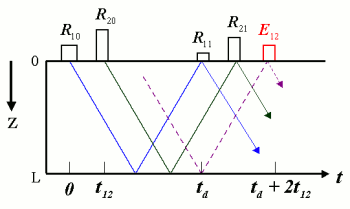Fig. 3: Schematic diagram showing generation of echo (E12) and reflection pulses (R11 and R21) as a result of input pulses (R10 and R20). The length of the sample is L and td is the roundtrip time .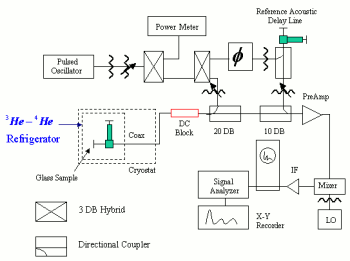Fig. 4: Schematic drawing of electronic instrumentation used for generation of input pulses and measurement of output signals .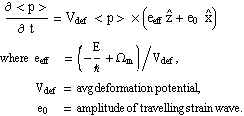In the above rotating frame, the applied acoustic pulse that is described as a traveling strain field, e = e0 cos(ω t - k z), appears stationary in time. If e0 >> eeff, then <p> precesses about the x-axis based on the above equation. Relaxation effects can be introduced into the above equations in analogy with Bloch equations [4,6]. Generally, we have the longitudinal relaxation time T1 and the homogeneous transverse relaxation time T2H ,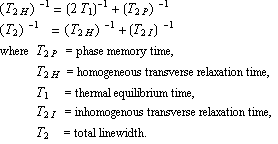Even at low temperature, the total linewidth T2 is very large due to the broad range of E of the two-level systems (i.e. T2I ∼ 0), which generally makes direct T2H measurement impossible. However, the generation and probing of phonon echoes provide a powerful tool for indirectly measuring T2H at these temperatures.

For glass at low temperature, T2H has a very different temperature dependence. This is because at lower temperatures the intrinsic width of the tunneling levels is less than the spectrum of the applied acoustic pulse  resulting in operation within the coherent regime where the phase as well as occupational number of the two-level systems is important. However, if the pulse width is increased or T2H decreased, we enter the incoherent regime where the pulse interaction with the medium can be solely described by occupational number of the two-levels. In order to observe phonon echoes, we must operate within the coherent regime.

In phonon echo experiment an important parameter is the area of the applied pulse ,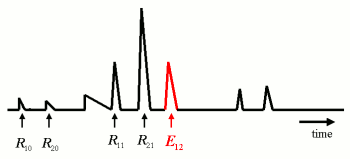Fig. 5: Sketch of envelope of phonon echo (E12) and reflection pulses (R11 and R21) resulting from the two input pulses (R10 and R20); the input pulses shown are heavily attenuated leakage pulses from the transducer . The pulses on the extreme right consist of multiple reflections and other echo signals [3,4].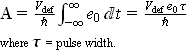The RHS equality is for the case of a rectangular pulse. The first pulse (R10) applied in the phonon echo experiment has an area of A1 = π / 2 and results in <p> rotating from its equilibrium position along the pz - axis to the px - py plane [Figure 2a]. The spread in frequencies of the two-level states, determined by the pulse spectrum ∼ 1 / τ, results in rapid dephasing in the px - py plane. On application of a second pulse (R20) with area A2 = π at t = t12, all two-level systems that have not lost their phase memory add up coherently to form an echo signal (E12) at t = 2 * t12 [Figure 2b]. Considering the valid assumption that the dephasing process occurs randomly, the echo intensity decays as Exp[-2 * t12 / T2H] . Hence, a plot of the amplitude of E12 vs t12 provides a measure of T2H.

It can be shown that the echo intensity has the following spatial and temperature dependence ,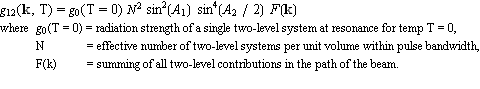The N2 dependence distinguishes phonon echoes as a coherent process where an ensemble of excited states are oscillating in phase . This is analogous to the "super-radiant state" that gives rise to photon echoes . On the other hand, the intensity in incoherent processes are generally characterized by a N dependence. In addition, the large size of the glass sample as compared to the wavelength in the acoustic experiment results in a spatial dependence (F(k)) of the intensity. F(k) is strongly peaked in the direction of k = 2 k2 - k1, where kX is the wavevector of the Xth pulse . In the phonon echo experiment considered, k1 = k2 and the phonon echo, thus, propagates in the forward direction. Figure 3 shows the input pulses R10 and R20 followed by the phonon echo E12. Also shown in Figure 3 are two reflection pulses R11 and R21 that are a result of total reflection of the input acoustic pulses at the surface of the sample .

Experimental Setup and Study of Phonon Echoes

The experiment setup required an efficient method for generating and detecting phonon pulses since the strain amplitudes associated with the necessary energy densities are very low. Furthermore, at energy densities slightly greater than that of thermal phonons, saturation of the tunneling states will occur . The glass sample used to generate phonon echoes was cooled to low temperatures (∼ 20 mk) with a 3He - 4He dilution refrigerator within a mixing chamber. The glass sample was a 0.635 mm cube of Suprasil W having a thin-film ZnO transducer (0.8 mm diameter) on one of two optically flat and parallel faces. On application through the transducer of two 0.68 Ghz, 100 ns duration excitation pulses R10 and R20 of the appropriate areas, the output signal was measured using the electronic circuit shown in Figure 4 (a sketch of the output signal envelope is shown in Figure 5). The electronic instrumentation consists of a pulsed oscillator whose output was split into two channel, one passing through the glass sample and the other serving as a reference channel. By comparing the output signal of the glass with the signal in the reference channel, the nonlinear response of the glass can be measured without worrying about the nonlinearities in the detection circuit. A more detailed description of the setup in Figure 4 is given in  and . The output signals measured by the signal detector are analogous to Figure 3 above. On repeating the experiment for various t12 values, the homogeneous transverse lifetime is found to be 14 us. One observation not mentioned above is the fact that the reflection pulse R11 and even the echo signal E12 has a higher amplitude than R11 . This is a result of saturation of the medium caused by R11. Another peculiarity not mentioned above which may not be very obvious from Figure 5 is that the output pulses R11, R21 and E12 are slightly broader than the input pulses. This broadening is related to self-induced transparency .

Conclusion

The generation and measurement of phonon echoes was described. This measurement allows the study of the dynamic characteristics of intrinsic two-level configurational states in glass at low temperatures. The phase memory (T2H) of the two-level systems at 20 mK was found to be 14 us. In addition to measurement of T2H using two pulse echo as described above, measurement of the longitudinal deformation potential is possible via the observation of multiple phonon echoes, and three pulse echoes enable the measurement of the longitudinal relaxation time (T1) . In fact, in the above experiment using three pulse echoes at 20mK, T1 was found to be ∼ 200us.

© 2007 Sunil Sandhu. The author grants permission to copy, distribute and display this work in unaltered form, with attribution to the author, for noncommercial purposes only. All other rights, including commercial rights, are reserved to the author.

References

 K. Fossheim, K. Kajimura, T. G. Kazyaka, R. L. Melcher, and N. S. Shiren, "Dynamic Polarization Echoes in Piezoelectric Powders," Phys. Rev. B 17, 964 (1978).

 R. W. Gould, "Echo Phenomena," Phys. Lett. 19, 447 (1965).

 B. Golding and J. H. Graebner, "Phonon Echoes in Glass," Phys. Rev. Lett. 37, 852 (1976).

 B. Golding, "Ultrasonic Properties of Glass at Ultra Low Temperatures," IEEE 1976 Ultrasonic Symposium Proceedings (IEEE, 1976), p. 692.

 J. H. Graebner and B. Golding, "Phonon Echoes in a Glass at Low Temperatures," Phys. Rev. B 19, 964 (1979).

 C. P. Slichter, Principles of Magnetic Resonance, 3rd edition (Springer, 1996).

 I. D. Abella, N.A. Kurnit, and S. R. Hartmann, "Photon Echoes," Phys. Rev. 141, 391 (1965).